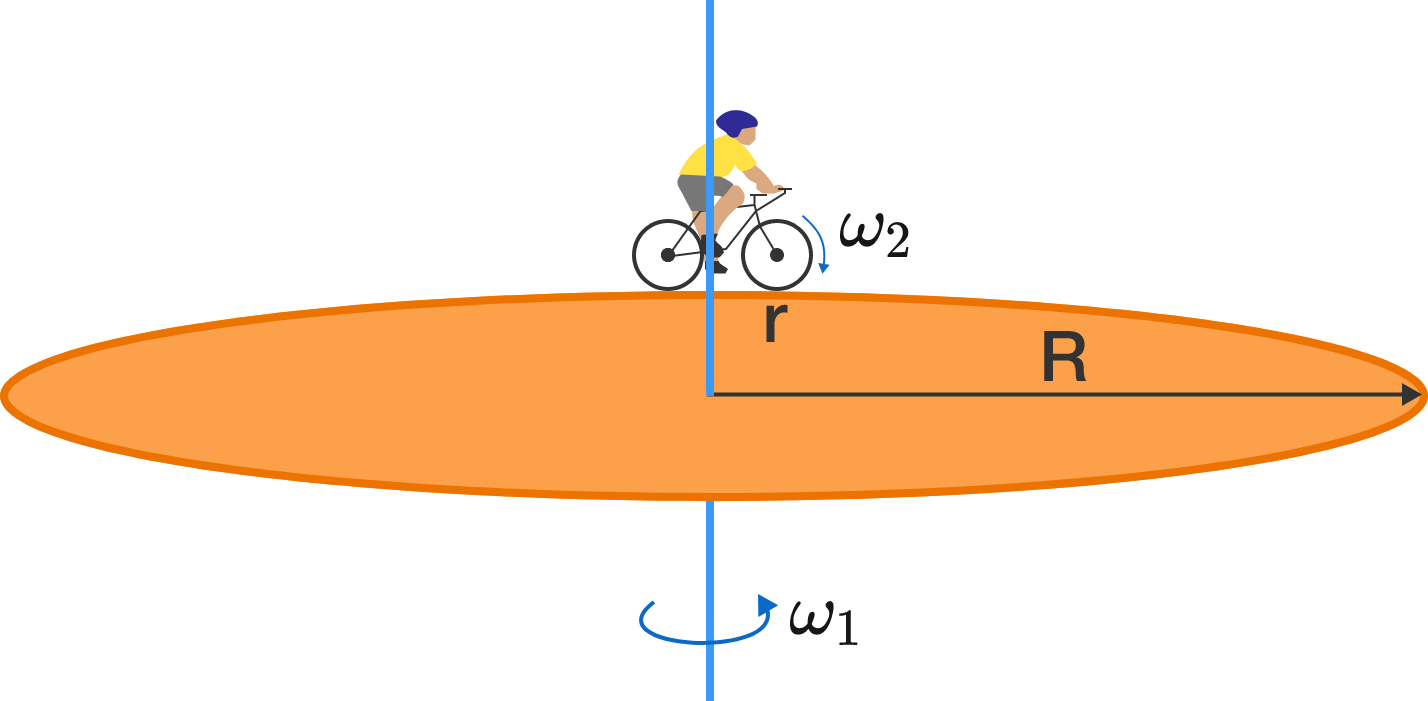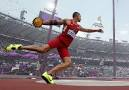Classical Mechanics

# Circular Motion - Level 2 ChallengesA cyclist is riding a bicycle of wheel radius $r$ along the edge of a rotating disk of radius $R\, (>r)$ in such a way that he appears to be stationary to a person standing on the ground.

If the tires of the bicycle don't slip on the disk (that is, the contact points of the wheel and the disk have equal velocities), then which will have a greater rotating speed?

Clarification: The "rotating speed" is the angular speed (or the magnitude of the angular velocity) given by the rate of change of $\theta$ with respect to the axis about which it is rotating:

$\omega = \dfrac{d\theta}{dt}$A bike moves at a rate of $\si{18\ \kilo\meter/\hour}$. The rear tire has a diameter of $\si{70\ \centi\meter}$ and has a toothwheel of diameter $\si{7\ \centi\meter}$. The sprocket (the gear that's turned by the pedals) has diameter $\si{20\ \centi\meter}$. Both gears are connected by the chain and there is no slippage between the chain and either gear. Under these conditions, how many times do the pedals revolve per minute?

In this matter, assume $\pi = 3$.

Old time mills for grinding wheat or corn into flour were often powered by a water wheel. Some water wheels had water falling over paddles, other water wheels dipped their paddles in a moving river, which as it flowed past turned the wheel. One particular mill has a water wheel with radius $3~\mbox{m}$ suspended above a river such that the bottom edge of the paddles are just in contact with and move with the river water. If the river flows at $0.5~\mbox{m/s}$ then what is the period of the spinning water wheel?Dominic is the star discus thrower on South's varsity track and field team. In last year's regional competition, Dominic whirled the $1.6\text{ kg}$ discus in a circle with a radius of $1.1\text{ m}$, ultimately reaching a speed of $52\text{ m/s}$ before launch. Determine the net force acting upon the discus in the moments before launch.A double-decker bus wants to take a turn safely, without tipping over. Which of the following will decrease the chance of the bus toppling?

×